# Transmission Line

Model transmission line

• Library:
• RF Blockset / Circuit Envelope / Elements

•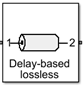## Description

Use the Transmission Line block to model delay-based, lumped, and distributed transmission lines. Mask dialog box options change automatically to accommodate model type selection.

## Parameters

expand all

### Main

Type of transmission line model, specified as one of these.

Transmission Line Types Description

`Delay-based and lossless`

Model transmission line with delay but no loss.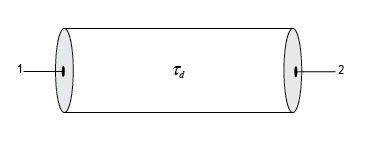`Delay-based and lossy`

Model transmission line with delay and loss.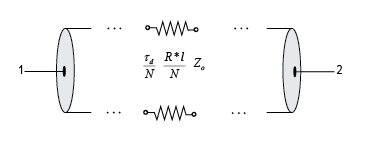`Lumped parameter L-section`

Model transmission line with RLGC L-sections.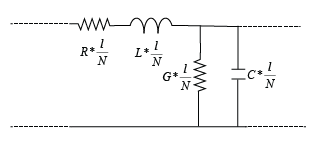`Lumped parameter Pi-section`

Model transmission line with RLGC pi-sections.`Coaxial`

Model transmission line as a coaxial transmission line. The cross section of a coaxial transmission line is shown in the following figure. Its physical characteristics include the radius of the inner conductor a, and the radius of the outer conductor b.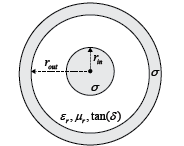`Coplanar waveguide`

Model transmission line as a coplanar waveguide. The cross section of a coplanar waveguide transmission line is shown in the following figure. Its physical characteristics include conductor width w, conductor thickness t, slot width s, substrate height d, and relative permittivity constant ε.`Microstrip`

Model transmission line as a standard, embedded, inverted, or suspended microstrip transmission line. The cross-sections of standard, embedded, inverted, and suspended microstrip transmission lines are shown here. The physical characteristics of such a transmission line include microstrip width w, microstrip thickness t, dielectric thickness d, and relative permittivity constant ε.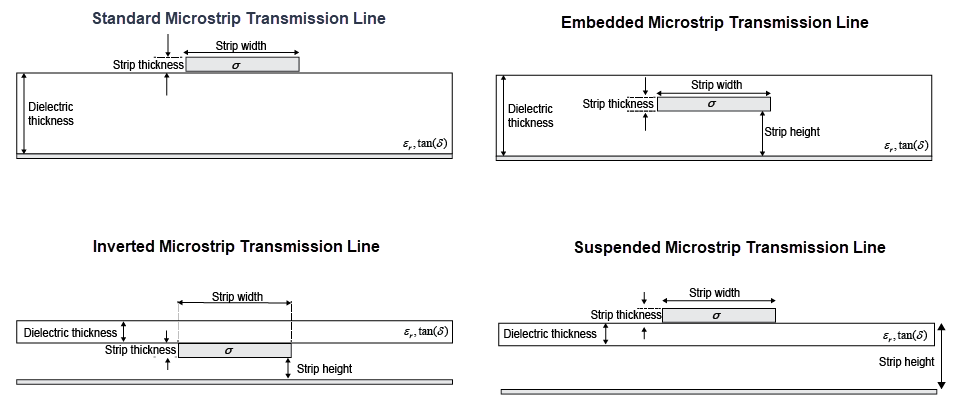`Stripline`

Model transmission line as stripline transmission line. The cross-section of a stripline transmission line is shown in this figure. Its physical characteristics include strip width w, strip thickness t, dielectric thickness h, and relative permittivity constant ε.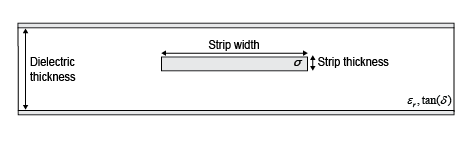`Two-wire`

Model transmission line as two-wire transmission line. The cross-section of a two-wire transmission line is shown in the following figure. Its physical characteristics include the radius of wires a, separation or physical distance between the wire centers S, and relative permittivity and permeability of the wires.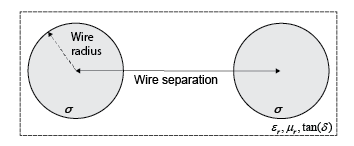```Parallel plate```

Model transmission line as a parallel-plate transmission line. The cross-section of a parallel-plate transmission line is shown in the following figure. Its physical characteristics include plate width w, and plate separation d. .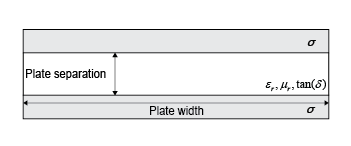```Equation based```

Model transmission line as an equation-based transmission line. The transmission line, which can be lossy or lossless, is treated as a two-port linear network.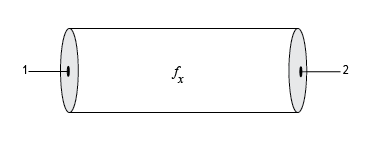`RLCG`

Model transmission line as an RLCG transmission line. This line is defined in terms of its frequency-dependent resistance, inductance, capacitance, and conductance. The transmission line, which can be lossy or lossless, is treated as a two-port linear network.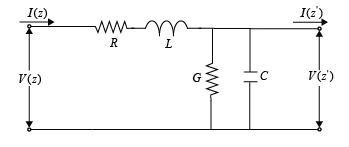Delay in the transmission line, specified as a real scalar in `s`, `ms`, `us`, or `ns`.

#### Dependencies

To enable this parameter, choose one of the following:

• `Delay-based and lossless` in Model type.

• `Delay-based and lossy` in Model type.

Impedance of the transmission line, specified as a real scalar in `Ohm`, `kOhm`, `MOhm`, or `GOhm`.

#### Dependencies

To enable this parameter, choose one of the following:

• `Delay-based and lossless`, `Delay-based and lossy`, or `Equation-based` in Model type.

• `Lumped parameter L-section` or ```Lumped parameter Pi-section``` in Model type and ```By characterisitc impedance and capacitance``` in Parameterization.

Resistance per unit length of the transmission line, specified as a positive scalar in `Ohm/m`, `kOhm/m`, `MOhm/m`, or `GOhm/m`.

#### Dependencies

To enable this parameter, choose one of the following:

• `Delay-based and lossy` or `RLCG` in Model type.

• `Lumped parameter L-section` or ```Lumped parameter Pi-section``` in Model type and ```By characterisitc impedance and capacitance``` in Parameterization.

Number of segments in the transmission line, specified as a positive scalar.

#### Dependencies

To enable this parameter, choose one of the following:

• `Delay-based and lossy` in Model type.

• `Lumped parameter L-section` or ```Lumped parameter Pi-section``` in Model type and ```By characterisitc impedance and capacitance``` or ```By inductance and capacitance``` in Parameterization.

Type of parameters to model segments in transmission line, specified as `By characterisitc impedance and capacitance` or `By inductance and capacitance`.

#### Dependencies

To enable this parameter, select ```Lumped parameter L-section``` or ```Lumped parameter Pi-section``` in Model type.

Capacitance per unit length of the transmission line, specified as a positive scalar in `F/m`, `mF/m`, `uF/m`, `nF/m`, or `pF/m`.

#### Dependencies

To enable this parameter, choose ```Lumped parameter L-section```, ```Lumped parameter Pi-section```, or `RLCG` in Model type.

Conductance per unit length of the transmission line, specified as a positive scalar in `S/m`, `mS/m`, `uS/m`, or `nS/m`.

#### Dependencies

To enable this parameter, choose ```Lumped parameter L-section```, ```Lumped parameter Pi-section```, or `RLCG` in Model type.

Inductance per unit length of the transmission line, specified as a positive scalar in `H/m`, `mH/m`, `uH/m`, or `nH/m`.

#### Dependencies

To enable this parameter, choose one of the following:

• `Lumped parameter L-section`, or ```Lumped parameter Pi-section``` in Model type and ```By inductance and capacitance``` in Parameterization.

• `RLCG` in Model type

Outer radius of coaxial transmission line, specified as a positive scalar in `m`, `cm`, `mm`, `um`, `in`, or `ft`.

#### Dependencies

To enable this parameter, choose `Coaxial` in Model type.

Inner radius of coaxial transmission line, specified as a positive scalar in `m`, `cm`, `mm`, `um`, `in`, or `ft`.

#### Dependencies

To enable this parameter, choose `Coaxial` in Model type.

Physical width of the conductor, specified as a positive scalar in `m`, `cm`, `mm`, `um`, `in`, or `ft`.

#### Dependencies

To enable this parameter, choose ```Coplanar waveguide``` in Model type.

Physical width of the slot, specified as a positive scalar in `m`, `cm`, `mm`, `um`, `in`, or `ft`.

#### Dependencies

To enable this parameter, choose ```Coplanar waveguide``` in Model type.

Select this parameter to add an infinite-bottom conductor to your coplanar waveguide transmission line.

#### Dependencies

To enable this parameter, set Model type to `Coplanar waveguide`.

Type of microstrip transmission line, specified as Standard, Embedded, Inverted, or Suspended.

#### Dependencies

To enable this parameter, set Model type to`Microstrip` .

Width of the microstrip transmission line, specified as a positive scalar in `m`, `cm`, `mm`, `um`, `in`, or `ft`.

#### Dependencies

To enable this parameter, set Model type to`Microstrip`.

Physical thickness of the conductor, specified as a nonnegative scalar in `m`, `cm`, `mm`, `um`, `in`, or `ft`.

#### Dependencies

To enable this parameter, choose ```Coplanar waveguide``` or `Microstrip` in Model type.

Strip height of the inverted, suspended, or embedded microstrip transmission line, specified as a positive scalar in `m`, `cm`, `mm`, `um`, `in`, or `ft`.

#### Dependencies

To enable this parameter, choose `Microstrip` in Model type and choose `Inverted`, `Suspended`, or `Embedded` in Structure.

Radius of the conducting wires of the two-wire transmission line, specified as a positive scalar in `m`, `cm`, `mm`, `um`, `in`, or `ft`.

#### Dependencies

To enable this parameter, choose `Two-wire` in Model type.

Physical distance between the conducting wires of the two-wire transmission line, specified as a positive scalar in `m`, `cm`, `mm`, `um`, `in`, or `ft`.

#### Dependencies

To enable this parameter, choose `Two-wire` in Model type.

Width of the parallel-plate transmission line, specified as a positive scalar in `m`, `cm`, `mm`, `um`, `in`, or `ft`.

#### Dependencies

To enable this parameter, choose `Parallel-plate` in Model type.

Thickness of the dielectric separating the plates, specified as a positive scalar in `m`, `cm`, `mm`, `um`, `in`, or `ft`.

#### Dependencies

To enable this parameter, choose `Parallel-plate` in Model type.

Propagation velocity of a uniform plane wave on the transmission line, specified as a positive scalar in meters per second

#### Dependencies

To enable this parameter, choose `Equation-based` in Model type.

Reduction in strength of the signal as it travels over the transmission line, specified as a positive scalar in meters per second

#### Dependencies

To enable this parameter, choose `Equation-based` in Model type.

Modeling frequencies, specified as a positive scalar or vector in `Hz`, `kHz`, `MHz`, or `GHz`.

#### Dependencies

To enable this parameter, choose `Equation-based` or `RLCG` in Model type.

Interpolation method used to calculate the values at the modeling frequencies, specified as `Linear`, `Spline`, or `Cubic`.

#### Dependencies

To enable this parameter, choose `Equation-based` or `RLCG` in Model type.

Conductivity of conductor, specified as a scalar in `S/m`, `mS/m`, `uS/m`, or `nS/m`.

#### Dependencies

To enable this parameter, choose `Coaxial`, ```Coplanar waveguide```, `Microstrip`, `Two-wire` or `Parallel-plate` in Model type.

Thickness of the dielectric on which the conductor resides, specified as a positive scalar in `m`, `cm`, `mm`, `um`, `in`, or `ft`.

Default values of the dielectric thickness of coplanar waveguide, standard, embedded, inverted, and suspended microstrip transmission lines are listed in this table.

Model TypeStructureDefault Dielectric Thickness in mm
`Coplanar waveguide`N.A.`0.635`
`Microstrip``Standard` and `Inverted``0.635`
`'Suspended'``0.3175`
`'Embedded'``1.37`

#### Dependencies

To enable this parameter, set Model type to `Microstrip` or ```Coplanar waveguide```.

Relative permeability of the dielectric, specified as a scalar.

#### Dependencies

To enable this parameter, set Model type to `Coaxial`, `Two-wire`, or `Parallel-plate`.

Relative permittivity of the dielectric, specified as a scalar.

#### Dependencies

To enable this parameter, set Model type to `Coaxial`, ```Coplanar waveguide```, `Microstrip`, `Two-wire`, or `Parallel-plate`.

Loss tangent of the dielectric, specified as a scalar.

#### Dependencies

To enable this parameter, set Model type to `Coaxial`, ```Coplanar waveguide```, `Microstrip`, `Two-wire`, or `Parallel-plate`.

Physical length of the transmission line or l, specified as a positive scalar in `m`, `cm`, `mm`, `um`, `in`, or `ft`.

#### Dependencies

To enable this parameter, choose one of the following:

• `Delay-based and lossy`, `Coaxial`, `Coplanar waveguide`, `Microstrip`, or `Two-wire`, `Parallel-plate`, `Equation-based`, or `RLCG` in Model type.

• `Lumped parameter L-section` or ```Lumped parameter Pi-section``` in Model type and ```By characterisitc impedance and capacitance``` or ```By inductance and capacitance``` in Parameterization.

Type of stub, specified as `Not a stub`, `Shunt`, or `Series`. See Parameter Calculations for Transmission Line with Stub.

#### Dependencies

To enable this parameter, choose `Coaxial`, ```Coplanar waveguide```, `Microstrip` `Two-wire`, `Parallel-plate`, `Equation-based`, or `RLCG` in Model type.

Type of termination for stub, specified as `Open` or `Short`.

#### Dependencies

To enable this parameter, choose `Series` or `Shunt` in Stub mode.

Select this parameter to internally ground and hide the negative terminals. To expose the negative terminals, clear this parameter. By exposing these terminals, you can connect them to other parts of your model.

By default, this option is selected.

### Modeling

Options to model S-parameters, specified as:

• `Frequency domain` – Computes the baseband impulse response for each carrier frequency independently. This technique is based on convolution. There is an option to specify the duration of the impulse response. For more information, see Compare Time and Frequency Domain Simulation Options for S-parameters.

• `Time domain (rationalfit)` – Computes the analytical rational model that approximates the whole range of the data.

For the Amplifier, Antenna, and S-Parameters blocks, the default value is `Time domain (rationalfit)`. For the Transmission Line block, the default value is ```Frequency domain```.

Select Automatically estimate impulse response duration to calculate impulse response duration automatically. Clear the selection to specify impulse response duration.

#### Dependencies

To enable this parameter, choose `Frequency domain` in Modeling options.

Manually specify impulse response duration, specified as a positive scalar in `s`, `ms`, `us`, or `ns`.

#### Dependencies

To enable this parameter, clear Automatically estimate impulse response duration.

Fitting options for rationalfit, specified as ```Share all poles```, `Share poles by columns`, or `Fit individually`.

For the Amplifier block, the default value is ```Fit individually```. For the S-parameters block and Transmission Line block, the default value is ```Share all poles```.

#### Dependencies

To enable this parameter, choose ```Time domain (rationalfit)``` in Modeling options.

Relative error acceptable in rationalfit output, specified as a real scalar in decibels.

#### Dependencies

To enable this parameter, choose ```Time domain (rationalfit)``` in Modeling options.

Shows values of Number of independent fits, Number of required poles, and Relative error achieved (dB).

When modeling using `Time domain`, the in `Visualization` tab plots the data defined in ```Data Source``` and the values in the `rationalfit` function.

#### Dependencies

To enable this parameter, choose ```Time domain (rationalfit)``` in Modeling options.

Note

Modeling tab is enabled for all transmission line types except `Delay-based and lossless`, `Delay-based and lossy`, ```Lumped parameter L-section```, and ```Lumped parameter pi-section```.

expand all

## Tips

• In general, blocks that model delay effects rely on signal history. You can minimize numerical error that occur due to a lack of signal history at the start of a simulation. To do so, in the Configuration Parameters dialog box Solver pane you can specify an Initial step size. For models with delay-based Transmission Line blocks, use an initial step size that is less than the value of the Delay parameter.

 Sussman-Fort, S. E., and J. C. Hantgan. “SPICE Implementation of Lossy Transmission Line and Schottky Diode Models.” IEEE Transactions on Microwave Theory and Techniques.Vol. 36, No.1, January 1988.

 Pozar, David M. Microwave Engineering. Hoboken, NJ: John Wiley & Sons, Inc., 2005.

 Gupta, K. C., Ramesh Garg, Inder Bahl, and Prakash Bhartia. Microstrip Lines and Slotlines, 2nd Edition, Norwood, MA: Artech House, Inc., 1996.

 Ludwig, Reinhold and Pavel Bretchko. RF Circuit Design: Theory and Applications. Englewood Cliffs: NJ: Prentice-Hall, 2000.

 True, Kenneth M. “Data Transmission Lines and Their Characteristics.” National Semiconductor Application Note 806, April 1992.